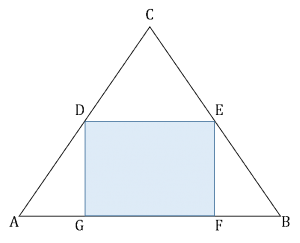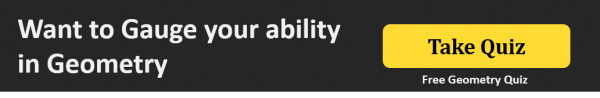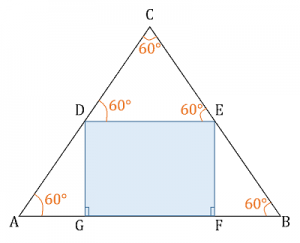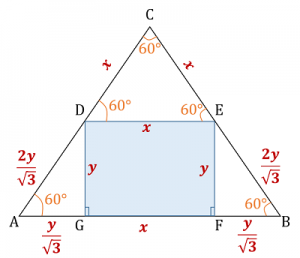GMAT Question of the Day - Daily to your Mailbox; hard ones only

 It is currently 12 Nov 2019, 16:49### GMAT Club Daily Prep

#### Thank you for using the timer - this advanced tool can estimate your performance and suggest more practice questions. We have subscribed you to Daily Prep Questions via email.

Customized
for You

we will pick new questions that match your level based on your Timer History

Track

every week, we’ll send you an estimated GMAT score based on your performance

Practice
Pays

we will pick new questions that match your level based on your Timer History

#### Not interested in getting valuable practice questions and articles delivered to your email? No problem, unsubscribe here.# Rectangle DEFG is inscribed in an equilateral triangle. . .

Author Message
TAGS:

### Hide Tags

e-GMAT RepresentativeV
Joined: 04 Jan 2015
Posts: 3134
Rectangle DEFG is inscribed in an equilateral triangle. . .  [#permalink]

### Show Tags00:00

Difficulty:75% (hard)

Question Stats:57% (02:22) correct43% (02:34) wrongbased on 129 sessions

### HideShow timer StatisticsRectangle DEFG is inscribed in an equilateral triangle as shown above. What is the area of triangle ABC?

(1) The area of rectangle DEFG is 8√3 square units
(2) The area of triangle AGD is 2√3 square units

To solve question of the week:Question of the Week

Take a stab at this fresh question from e-GMAT. Post your analysis below.

Official Solution to be provided after receiving some good analyses._________________

Originally posted by EgmatQuantExpert on 08 Nov 2016, 05:13.
Last edited by EgmatQuantExpert on 07 Aug 2018, 06:59, edited 2 times in total.
InternJoined: 16 Jul 2016
Posts: 2
Re: Rectangle DEFG is inscribed in an equilateral triangle. . .  [#permalink]

### Show Tags

Is the answer option c ie both 1 and 2 are required

Sent from my Lenovo A7010a48 using GMAT Club Forum mobile app
ManagerB
Joined: 27 Aug 2015
Posts: 86
Re: Rectangle DEFG is inscribed in an equilateral triangle. . .  [#permalink]

### Show Tags

St 1 - Gives us area of rect but there can be n number of rectangles so we cant fnd any side of triangle - NS
St2 - Again it gives us the area of small triangles. With this we can find one side of rectangle and sides of small triangles but we can have diff sizes of such triangles.
Combining we can find sides of square , then sides of small triangles. With these we can find the side of triangle- so Suff.
ManagerB
Joined: 28 Jun 2016
Posts: 206
Concentration: Operations, Entrepreneurship
Re: Rectangle DEFG is inscribed in an equilateral triangle. . .  [#permalink]

### Show Tags

Area of equilateral triangle =sqrt(3)*AB^2 / 4

So AB=?

Statement 1:

DG*GF=8*sqrt(3)

Insufficient

Statement 2:

1/2*AG*DG=2*sqrt(3)

We know ADG is a 30-60-90 triangle

So AG*AG*sqrt(3)=4*sqrt(3)

AG=2

DG=2*sqrt(3)

Insufficient

Statement 1&2:

2*sqrt(3)*GF=8*sqrt(3)

GF=4

We know that

BF=AG=2

Therefore AB= 2+2+4=8

Area= sqrt(3)*8^2 /4 = 16*sqrt(3)

Sufficient

C

Sent from my iPhone using GMAT Club Forum mobile app
InternJoined: 22 Mar 2016
Posts: 2
Location: India
GPA: 3.8
Re: Rectangle DEFG is inscribed in an equilateral triangle. . .  [#permalink]

### Show Tags

Statement1: insufficient because we can have multiple values of sides of rectangle DEFG.
Statement2: Insufficient again.
Combining:
Draw a perpendicular CP that intersects DE at O and AB at P.
We can also see that area of triangle ADG is 1/4 the area of DEFG.
Triangles AGD and PGD can be proved congruent.
DE parallel to AB.
DE=1/2AB (AG=GP=PF=FB, DE. DE=GP+PF, AB=AG+GP+PF+FB)
by mid point theorum,points D and E are mid points of AC and BC respectively.
so all triangles have equal area i.e.
Thanks. Post Kudos if you like.
e-GMAT RepresentativeV
Joined: 04 Jan 2015
Posts: 3134
Re: Rectangle DEFG is inscribed in an equilateral triangle. . .  [#permalink]

### Show Tags

2
Solution

Hey,

Please find below the official solution.

• We are given that $$ABC$$ is an equilateral triangle.
o This means, each angle of this triangle is $$60^o$$.

• Also, $$DEFG$$ is a rectangle.
o Hence each angle of this rectangle is $$90^o$$.

• Since the opposite sides of a rectangle are parallel, $$DE || GF$$, and therefore, $$DE || AB$$.
o So, $$∠ CDE = ∠ CAB = 60^o$$ (corresponding angles)
o Similarly, $$∠ CED = ∠ CBA = 60^o$$.• Now, let’s assume that side $$GF = x$$ units and side $$GD = y$$ units.

So, expressing all the unknown lengths in terms of x and y, we get:Note:

• Since Triangle $$AGD$$ is a $$30^o-60^o-90^o$$ triangle, the ratio of the sides are $$AG : DG : AD = 1 : √3 : 2$$
• Thus if $$DG = y$$, then $$AG = y/√3$$ and $$AD = 2y/√3$$
• And we can conclude the same for the sides of triangle $$EFB$$.

From the above diagram, we can infer that –
• The Sides of an equilateral triangle $$ABC = x+2y/√3$$

Thus, in order to find the area of the triangle, we need to know the value of x and y.

Analyze Statement 1 independently

• The area of rectangle $$DEFG$$ is $$8√3$$ square units
o That is, $$xy = 8√3$$ . . . (1)
o Multiple values of x and y will satisfy this equation.

Therefore statement 1 is not sufficient to arrive at a unique answer.

Analyze Statement 2 independently

• The area of triangle $$AGD$$ is $$2√3$$ square units

Thus, we can write -
• $$1/2*AG*GD=2√3$$
• $$1/2*y/√(3 )*y=2√3$$
• $$y^2=2^2×3$$
• $$y=2√3$$ . . . (2)
• But we don’t know the value of x yet.

Therefore, statement 2 is not sufficient to arrive at a unique answer.

Analyze by combining statement 1 and 2

• Put the value of y from equation (2) in equation (1) and we will get: $$x = 4$$
• Since we now know the value of x and y, we can find the area of triangle.

Therefore both statement 1 and 2 are required to answer the question.

Hence the correct Answer is C

Thanks,
Saquib
Quant Expert
e-GMAT
_________________
Non-Human UserJoined: 09 Sep 2013
Posts: 13566
Re: Rectangle DEFG is inscribed in an equilateral triangle. . .  [#permalink]

### Show Tags

Hello from the GMAT Club BumpBot!

Thanks to another GMAT Club member, I have just discovered this valuable topic, yet it had no discussion for over a year. I am now bumping it up - doing my job. I think you may find it valuable (esp those replies with Kudos).

Want to see all other topics I dig out? Follow me (click follow button on profile). You will receive a summary of all topics I bump in your profile area as well as via email.
_________________Re: Rectangle DEFG is inscribed in an equilateral triangle. . .   [#permalink] 18 Oct 2019, 14:57
Display posts from previous: Sort by

# Rectangle DEFG is inscribed in an equilateral triangle. . .